Lecture 19 - PHY294H

Recommended problems for Lecture 19: 29.47, 29.55, 29.54

Two remarks:

(i) Magnetic field lines come out of the N pole and go into the S pole.

(ii) North geographic pole is close to the South magnetic pole.

A current carrying wire in a magnetic field

We have learned that a moving charge experiences a force which is equal to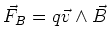. Since current flow corresponds to moving charges, a current carrying wire should also experience a force when it is placed in a perpendicular magnetic field. The force on a straight wire carrying current I is easily derived from the magnetic force. The velocity which contributes to current is the drift velocity, vd. The current density is given by nq q vd and the current is I= jA = A nqqvd. The force on each charge is then, on average,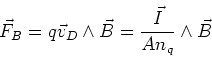(1)

The force on the wire is proportional to the number of charge carriers are travelling through the magnetic field, i.e. N = nq V = nq A lwhere l is the length of the wire. We thus have,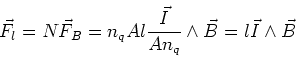(2)

The direction of the current flow can be kept in either I or in the length l. If we have a small piece of wire in a magnetic field we may then write,(3)

Here we have assigned the direction of current flow to the vector direction of the wire. The direction of the force is given by the right hand rule, as is the case for the magnetic force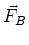.

If we have a wire which changes direction, then the total force on the wire is a vector sum of terms of the form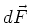. We have to integrate these terms over the length of the wire, that is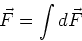(4)

Long straight wire

If we place a long straight wire of length L and at angle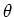to a constant magnetic field (B), then the force on the wire is given by,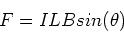(5)

Its direction if given by the right hand rule.

FGT 29.45

The magnetic force on the wire is ILB down the page. At equilibrium this is equal to the force in the springs, F = 2 k y. Equating these two forces, we have,

 y = ILB/2k (6)

Magnetic force on current loops

Current loop in a magnetic field

Consider a rectangular loop, which has its long sides with length b and its short sides with length a, and which carries current I. This loop is mounted on an insulating axis of rotation. This axis is perpendicular to the direction of the magnetic field, which is assumed to be uniform. We want to find the force on this loop as a function of the angle between the loop and the direction of the magnetic field. We take the angle between the normal to the loop and the direction of the magnetic field to be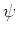and we define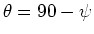. Let us take the long sides of the loop to be parallel to the axis of rotation and the short axes of the loop to be perpendicular to the axis of rotation. The first result is that if the magnetic field is constant, there is no net force on the loop. We demonstrate this by treating first the two long sides of the loop:

The force on each of these sides is F = I b B and F=-IbB. The forces on the short sides is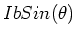and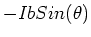. There is thus no net force on the loop.

Altough there is no net force on the loop, there is a net torque. The torque is defined as,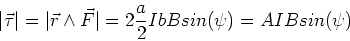(7)

The direction of the torque is along the axis of rotation. This result is a special case of a general result which can be written as,(8)

provided we define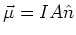to be the magnetic moment of the current loop and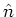to be the unit normal of the current loop The direction of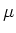is given by the right hand rule applied to the current flow. It is simple to generalize this result to the case where the loop has N turns. In that case,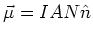. Notice that Eq. (8) looks the same as the expression which we found for an electric dipole in an electric field (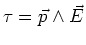).

FGT 29.54

In this problem we cannot usebecause the current does not go around the loop. The dipole moment formula only works for current loops. Instead, this is a current divider. The current V1=V2, so I1R1 = I2R2. We also have I=I1+I2, so the currents I1 and I2 are given by,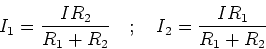(9)

The torque on the loop is then given by,(10)